Next: 2.3 Results Up: 2.2 Mapping to a Previous: 2.2.2 Equivalence to a

## 2.2.3 Calculation of physical quantities

Once the mapping between the SIKM (2.1) and the effective RLM (2.8) has been established, one can readily calculate physical quantities for the Kondo model. One complication arises from the fact that operators of the original SIKM have to be transformed by a unitary transformation analogous to (2.2). In the language of the effective resonant level model they will thus in general correspond to more complicated many-particle operators. Since the intention of this chapter is to demonstrate the potential of our mapping in a pedagogical setting, we will concentrate on two quantities that remain simple under these transformations: i) the-component of the spin operator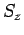, which becomes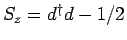, and ii) the Hamiltonian itself.

From the latter we obtain the internal energy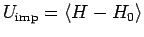and the Sommerfeld coefficient,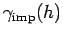. A straightforward calculation in the noninteracting RLM yields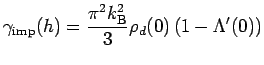(2.17)

wheredenotes the derivative ofandis the principal value integral. Hereis the impurity orbital density of states of the RLM(2.18)

The result (2.17) forhas some interesting implications. First, becauseis connected to, it is apparent that the low-energy excitations in the system are controlled by spin degrees of freedom, a well-known feature of the Kondo physics. However, in our approach this result can be read off directly from equ. (2.17). Second,, i.e. we obtain the correct scaling behavior fordirectly from the behavior of. There is, however, a nontrivial correction coming from the factor in parenthesis in (2.17). Note that forconst. this correction is one, but for the strongly-dependentin Fig. 2.1 it is of the order of two. As we will demonstrate later, this difference is directly responsible for obtaining the correct Wilson ratio in our approach.

From the mappingit is easy to calculate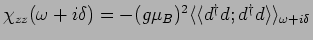. Since the correlation function has to be evaluated within the RLM, one obtains for the imaginary part at(2.19)

Again, this result provides direct access to an interpretation of the behavior ofin terms of the physics of the resonant level model.Next: 2.3 Results Up: 2.2 Mapping to a Previous: 2.2.2 Equivalence to a
© Cyrill Slezak